Short Answers - Polynomials - Practice

# Short Answers - Polynomials - Practice Notes - Class 9

## Document Description: Short Answers - Polynomials - Practice for Class 9 2022 is part of Class 9 preparation. The notes and questions for Short Answers - Polynomials - Practice have been prepared according to the Class 9 exam syllabus. Information about Short Answers - Polynomials - Practice covers topics like and Short Answers - Polynomials - Practice Example, for Class 9 2022 Exam. Find important definitions, questions, notes, meanings, examples, exercises and tests below for Short Answers - Polynomials - Practice.

Introduction of Short Answers - Polynomials - Practice in English is available as part of our Class 9 preparation & Short Answers - Polynomials - Practice in Hindi for Class 9 courses. Download more important topics, notes, lectures and mock test series for Class 9 Exam by signing up for free. Class 9: Short Answers - Polynomials - Practice Notes - Class 9
 1 Crore+ students have signed up on EduRev. Have you?

Q.1. Write the numerical co-efficient and degree of each term of: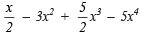Sol.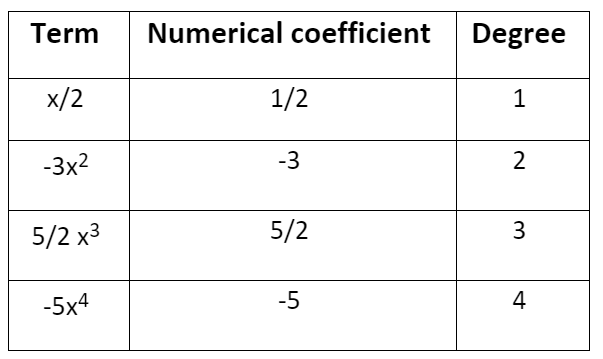Q.2. Find the remainder when x3 – ax2 + 4x – a is divided by (x – a).
Sol.

p(x) = x– ax2 + 4x – a
(x – a) = 0
► x = a
∴ p(a) = (a)3 – a(a)2 + 4(a) – a = a3 – a+ 4a – a = 4a – a = 3a
∴ The required remainder = 3a.

Q.3. When the polynomial kx3 + 9x2 + 4x – 8 is divided x + 3, then a remainder 7 is obtained.
Find the value of k.
Sol.

Here, p(x) = kx3 + 9x+ 4x – 8
Since, Divisor = x + 3
∴ x + 3 = 0
► x = –3
∴ p(–3) = 7
► k(–3)3 + 9(–3)+ 4(–3) – 8 = 7
► –27k + 81 – 12 – 8 = 7
► –27k = 7 – 81 + 12 + 8
► –27k = 27 – 81
► –27k = –54
► k= -(54/27) = 2
Thus, the required value of k = 2.

Q.4. (a) For what value of k, the polynomial x2 + (4 – k)x + 2 is divisible by x – 2?
(b) For what value of ‘m’ is x3 – 2mx2 + 16 is divisible by (x + 2)?

Sol.
(a) Here p(x) = x+ 4x – kx + 2
If p(x) is exactly divisible by x – 2, then p(2) = 0
i.e. (2)2 + 4(2) – k(2) + 2 = 0
► 4 + 8 – 2k + 2 = 0
► 14 – 2k = 0
► 2k = 14
► k= 14/2 = 7

Thus, the required value of k is 7.

(b) Here, p(x) = x3 – 2mx2 + 16
∴ p(–2) = (–2)3 –2(–2)2m + 16
= –8 –8m + 16
= –8m + 8
Since, p(x) is divisible by x + 2
∴ p(–2) = 0
or –8m + 8 = 0
► m = 1

Q.5. Factorize x2 – x – 12.
Sol.

We have  x2 – x – 12

= x– 4x + 3x – 12
= x(x – 4) + 3(x – 4)
= (x – 4)(x + 3)
Thus, x– x – 12 = (x – 4)(x + 3)

Q.6. If x + (1/2)x  = 5, then find the value of x2 +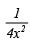Sol.
We have x + (1/2)x  = 5

Squaring both sides, we get: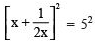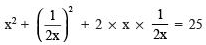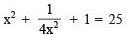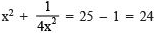Thus, the required value of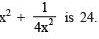Q.7. Check whether (x – 1) is a factor of the polynomial x3 – 27x2 + 8x + 18.
Sol.

Here, p(x) = x– 27x2 + 8x + 18, (x – 1) will be a factor of p(x) only if (x – 1) divides p(x) leaving a remainder 0.
For  x – 1 = 0
► x = 1
∴ p(1) = (1)3 – 27(1)2 + 8(1) + 18
= 1 – 27 + 8 + 18
= 27 – 27
= 0
Since, p(1) = 0
∴ (x – 1) is a factor of p(x).
Thus, (x – 1) is a factor of x3 – 27x2 + 8x + 18.

Q.8. Find the remainder when f(x) =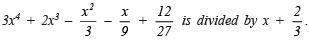Sol.
Here f(x) =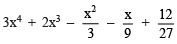Divisor = x+(2/3)

since, x+(2/3) = 0 ⇒ x = -2/3

∴ Remainder = f (-2/3)

i.e. Remainder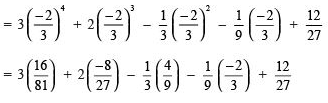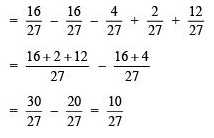Thus, the required remainder =  (10/27)

Q.9. Find the value of k, if (x – k) is a factor of x6 – kx5 + x4 – kx3 + 3x – k + 4.
Sol.

Here, p(x) = x6 – kx5 + x– kx3 + 3x – k + 4
If (x – k) is a factor of p(x), then p(k) = 0
i.e (k)6 – k(k5) + k4 – k(k3) + 3k – k + 4 = 0
► k– k6 + k4 – k4 + 3k – k + 4 = 0
► 2k + 4 = 0
► 2k = – 4
► k = (-4/2) = –2
Thus, the required value of k is –2.

Q.10. Factorize: 9a2 – 9b2 + 6a + 1
Sol.

9a2 – 9b2 + 6a + 1
= [9a2 + 6a + 1] – 9b
= [(3a)2 + 2(3a)(1) + (1)2] – (3b)
= (3a + 1)2 – (3b)2
= [(3a + 1) + 3b][(3a + 1) – 3b]    {using x– y2 = (x – y)(x + y)}
= (3a + 1 + 3b)(3a + 1 – 3b)

Q.11. Find the value of x3 + y3 – 12xy + 64, when x + y = –4.
Sol.

x3 + y– 12xy + 64
= (x)3 + (y)3 + (4)– 3(x)(y)(4)
= [x2 + y2 + 42 – xy – y. 4 – 4 .  x](x + y + 4)
= [x2 + y2 + 16 – xy – 4y – 4x][x + y + 4]                    ...(1)
Since, x + y = –4 ∴ x + y + 4 = 0                                 ...(2)

From (1) and (2), we have x3 + y– 12xy + 64
= [x+ y2 + 16 – xy – 4y – 4x] = 0
Thus, x3 + y3 – 12xy + 64 = 0.

The document Short Answers - Polynomials - Practice Notes - Class 9 is a part of Class 9 category.
All you need of Class 9 at this link: Class 9
 Use Code STAYHOME200 and get INR 200 additional OFF

Track your progress, build streaks, highlight & save important lessons and more!

,

,

,

,

,

,

,

,

,

,

,

,

,

,

,

,

,

,

,

,

,

;